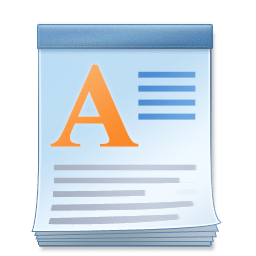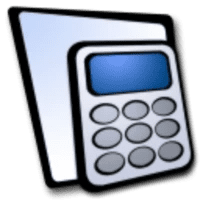()

Microsoft Mathematics, also known as Microsoft Math, is software created to help students learn math. This is done by using the available features to understand how and when it is appropriate to use functions across many different mathematics areas.

## Software description

Developed by Microsoft in 2008, Microsoft Mathematics was first available as a part of the Microsoft Student bundle. It ran only on Windows until 2015 when it was updated to run as a web platform and a mobile app. In it’s fourth version it is no longer supported with active updates, though it is still a useful tool for students.

### Features

Microsoft Mathematics has several useful features you can use to help in many areas of math and even in some areas of science. There are a few areas that you should know about to effectively use the program. These include the clipboard, numbers and angles, input, and tools categories to choose from to maximize the program.

The main dropdowns are for the clipboard, numbers and angels function, input, and tools functions to assist you in solving problems. They clipboard words the same way as in Microsoft Word and input recognizes how you enter information. Numbers and angles can switch between real and imaginary numbers and whether the equations will be in degrees, radians, or gradians. Tools where you go to get the step-by-step instructions .

### Using Tools

Several different tools are used to solve different equations. These are the bread and butter of the program and what helps students learn how to solve problems on their own.

### Equation Solver

Enter the equation you need to solve. Then, with one click, you get step-by-step instructions on solving problems. Also, you can see other ways to solve or a graph of the problem.

### Formulas and Equations

Choose from categories to solve from and then, with one click, solve or see the graphic solution to the problem. Choose from:

• Algebra
• formulas and equations
• geometry
• physics
• statistics
• chemistry
• Law of Exponents
• trigonometry
• properties of logarithms
• constants

### Triangle Solver

This will enable you to calculate the length of the sides of any triangle. It can also help in solving the measures of all the angles in a triangle and with the Pythagorean Theorem.

### Unit Converter

This tool will easily convert all types of measurements applicable in both math and science professions and industries. You can convert almost anything, including:

• length
• mass
• volume
• area
• width
• weight
• pressure
• temperature
• power
• velocity
• time
• force
• energy

### Graphing Tools

This is a part of the equation solving function. When you input a problem there will be an option inblue in order to graph one or both sides of an equation.

### Types of Math Included

Microsoft Math can assist you in almost every area of math. These include:

• Statistics
• Linear algebra
• Standard Math
• Trigonometry
• Geometry
• Calculus

Though it is no longer supported by or available in the Microsoft website, you can still find Microsoft Math as a mobile app or as a plugin for Microsoft Word or OneNote. This lets you do math right inside those applications.

### A Useful Problem Solver

Microsoft Mathematics is a exceptionally useful program if you are looking for some help solving equations. It will allow you to learn and understand the way to solve more complicated math problems. It is a great tool to get you solving these problems all on your own.

### Top Softwares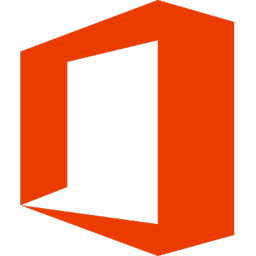#### Microsoft Office

Microsoft Office is the complete, cross-platform, cross-device solution for the...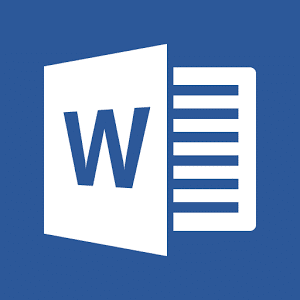#### Microsoft Word

Microsoft Word is an application that can be found in...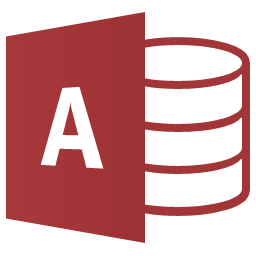#### Microsoft Access

Microsoft Access is a powerful integrated database management system (DBMS)...#### Kindle App

The Kindle app is an app for everyone who likes...